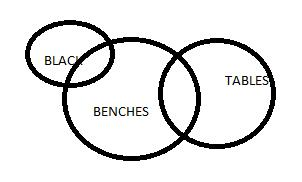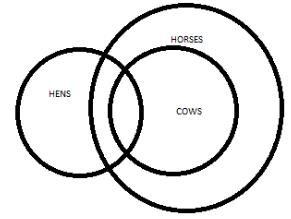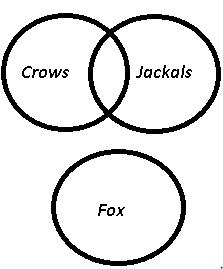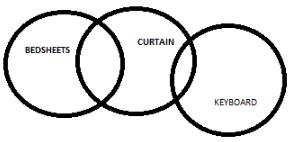Courses

# Test: Syllogism- 3

## 20 Questions MCQ Test Logical Reasoning for CLAT | Test: Syllogism- 3

Description
This mock test of Test: Syllogism- 3 for CLAT helps you for every CLAT entrance exam. This contains 20 Multiple Choice Questions for CLAT Test: Syllogism- 3 (mcq) to study with solutions a complete question bank. The solved questions answers in this Test: Syllogism- 3 quiz give you a good mix of easy questions and tough questions. CLAT students definitely take this Test: Syllogism- 3 exercise for a better result in the exam. You can find other Test: Syllogism- 3 extra questions, long questions & short questions for CLAT on EduRev as well by searching above.
QUESTION: 1

### Directions : In each question below are given two Statements: followed by two conclusion numbered I and II. You have to take the two given statement to be true even if they seem to be at variance with commonly known facts and then decide which of the given Conclusions: logically follows from the two given statement, disregarding commonly know facts. Statements:                       Some benches are tables.              No table is black. Conclusions:                     I. Some tables are benches.              II. No bench is black.

Solution:some tables are benches, and some benches or all benches can be black.

QUESTION: 2

Solution:
QUESTION: 3

### Directions : In each question below are given two Statements: followed by two conclusion numbered I and II. You have to take the two given statement to be true even if they seem to be at variance with commonly known facts and then decide which of the given Conclusions: logically follows from the two given statement, disregarding commonly know facts. Statements:                                    All pendrives are chalks.              All chalks are computers. Conclusions:                     I. All computers are pendrives.             II. All pendrives are computers

Solution:
QUESTION: 4

Directions : In each question below are given two Statements: followed by two conclusion numbered I and II. You have to take the two given statement to be true even if they seem to be at variance with commonly known facts and then decide which of the given Conclusions: logically follows from the two given statement, disregarding commonly know facts.

Statements:

Some children are flowers.

Some flowers are novels.

Conclusions:

I. Some children are novels.

II. No child is a flower.

Solution:
QUESTION: 5

Directions : In each question below are given two Statements: followed by two conclusion numbered I and II. You have to take the two given statement to be true even if they seem to be at variance with commonly known facts and then decide which of the given Conclusions: logically follows from the two given statement, disregarding commonly know facts.

Statements:

Some hens are cows.

All cows are horses.

Conclusions:

I. Some horses are hens.

II. Some hens are horses

Solution:

The correct option is D.

Both the conclusions can be drawn from the given statement.QUESTION: 6

Directions : In each question below are given two Statements: followed by two conclusion numbered I and II. You have to take the two given statement to be true even if they seem to be at variance with commonly known facts and then decide which of the given Conclusions: logically follows from the two given statement, disregarding commonly know facts.

Statements:

Some poets are producers.

Some producers are trees.

Conclusions:

I. Some poets are trees.

II. Some trees are producers.

Solution:
QUESTION: 7

Directions : In each question below are given two Statements: followed by two conclusion numbered I and II. You have to take the two given statement to be true even if they seem to be at variance with commonly known facts and then decide which of the given Conclusions: logically follows from the two given statement, disregarding commonly know facts.

Statements:

No pole is a house.

Conclusions:

II. Some houses are poles.

Solution:
QUESTION: 8

Directions : In each question below are given two Statements: followed by two conclusion numbered I and II. You have to take the two given statement to be true even if they seem to be at variance with commonly known facts and then decide which of the given Conclusions: logically follows from the two given statement, disregarding commonly know facts.

Statements:

All novels are pencils.

All pencils are flowers.

Conclusions:

I. All novels are flowers

II. Some pencils are not book.

Solution:
QUESTION: 9

Directions : In each question below are given two Statements: followed by two conclusion numbered I and II. You have to take the two given statement to be true even if they seem to be at variance with commonly known facts and then decide which of the given Conclusions: logically follows from the two given statement, disregarding commonly know facts.

Statements:

Some crows are jackals.

No fox is crow.

Conclusions:

I. Some jackals are foxes.

II. Some jackals are not foxes.

Solution:Conclusion:

I. Some jackals are foxes. = may or may not be true

II. Some jackals are not foxes. = may or may not be true

QUESTION: 10

Directions : In each question below are given two Statements: followed by two conclusion numbered I and II. You have to take the two given statement to be true even if they seem to be at variance with commonly known facts and then decide which of the given Conclusions: logically follows from the two given statement, disregarding commonly know facts.

Statements:

Some curtains are bed sheets.

Very few keyboards are curtains.

Conclusions:

I. Some bed sheets are keyboards.

II. No curtains are keyboard.

Solution:

The correct option is CQUESTION: 11

Directions : In each question below are given two Statements: followed by two conclusion numbered I and II. You have to take the two given statement to be true even if they seem to be at variance with commonly known facts and then decide which of the given Conclusions: logically follows from the two given statement, disregarding commonly know facts.

Statements:

All table lamps are electric goods.

Conclusions:

Solution:

C is the correct option. Neither of the conclusions follows because If all radios are electric goods and all table lamps are electric goods there is a possibility that radios might overlap table lamps but here the conclusions are SOME radios are table lamps and SOME table lamps are radios which we can not say clearly.

QUESTION: 12

Directions : In each question below are given two Statements: followed by two conclusion numbered I and II. You have to take the two given statement to be true even if they seem to be at variance with commonly known facts and then decide which of the given Conclusions: logically follows from the two given statement, disregarding commonly know facts.

Statements:

Men are sinners.

Saints are men.

Conclusions:

I. Saints are sinners.

II. Sinners are saints

Solution:
QUESTION: 13

Directions : In each question below are given two Statements: followed by two conclusion numbered I and II. You have to take the two given statement to be true even if they seem to be at variance with commonly known facts and then decide which of the given Conclusions: logically follows from the two given statement, disregarding commonly know facts.

Statements:

Conclusions:

I. This thief is a man.

II. Some men are thieves.

Solution:

D is the correct option.in this question statement implies that a graduate is a man and a man is a thief. The assumption is this thief would be man or some thieves would be man. So both conclusions are follows.

QUESTION: 14

Directions : In each question below are given two Statements: followed by two conclusion numbered I and II. You have to take the two given statement to be true even if they seem to be at variance with commonly known facts and then decide which of the given Conclusions: logically follows from the two given statement, disregarding commonly know facts.

Statements:

All gases are solids.

All solids are liquids.

Conclusions:

I. All gases are liquids.

II. At least some liquids are solids.

Solution:
QUESTION: 15

In each of the questions below are given three statements followed by three or more conclusions. You have to take the given statements to be true even if they seem to be at variance with the commonly known facts.

Statements:

Some ducks are canes.

Some canes are books.

All books are lanterns.

Conclusions:

I.   Some lanterns are canes.

II.  Some lanterns are ducks.

III. All canes are lanterns.

IV.  All ducks are lanterns.

Solution:
QUESTION: 16

In each of the questions below are given three statements followed by three or more conclusions. You have to take the given statements to be true even if they seem to be at variance with the commonly known facts.

Statements:

All markers are black.
All needles are black.
All toys are black.

Conclusions:

I. Some needles are markers.

II.  Some toys are markers.

III. All markers are toys.

IV. All black are markers.

Solution:

The correct answer is D as none of the options given is correct and by drawing the vein diagram .

QUESTION: 17

In each of the questions below are given three statements followed by three or more conclusions. You have to take the given statements to be true even if they seem to be at variance with the commonly known facts.

Statements:

All stores are tents.
Some tents are stones.
All stones are walls.

Conclusions:

I.   Some walls are stores.

II.    Some walls are tents.

III. All stones are stores.

IV.   All walls are stores.

Solution:
QUESTION: 18

In each of the questions below are given three statements followed by three or more conclusions. You have to take the given statements to be true even if they seem to be at variance with the commonly known facts.

Statements:

Some bricks are gates.
Some gates are roof.
All wheels are bricks.

Conclusions:

I. Some wheels are gates.

II.  No gate is wheel

III.  Some gates are bricks.

IV.  All roof are bricks.

Solution:

D is the correct option. a and b both are possible conclusions.. either a or b and it follows 3 as some bricks are gates therefore there is a clear probability of some gates are bricks.

QUESTION: 19

In each of the questions below are given three statements followed by three or more conclusions. You have to take the given statements to be true even if they seem to be at variance with the commonly known facts.

Statements:

All desks are benches.
No bench is chair.

Conclusions:

II.   No chair is desk.

Solution:

B is the correct option as I and II follow the statement. III and IV don't follow as if all desks are benches and no bench is chair, so none of the chairs can be a desk and also if all chairs are road so some roads can be chairs.

QUESTION: 20

In each of the questions below are given three statements followed by three or more conclusions. You have to take the given statements to be true even if they seem to be at variance with the commonly known facts.

Statements:

All chains are cycles.
Some cycles are wheels.
All wheels are mirrors.

Conclusions:

I.   Some cycles are mirrors.

II.   Some wheels are cycles.

III. Some mirrors are cycles.

IV.   All chains are wheels.

Solution: